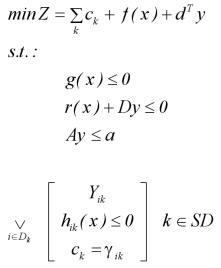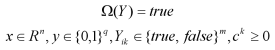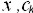are continuous variables,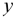are binary variables (0-1),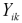are Boolean variables, to establish whether a disjunction term is true or false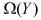logic relationships between Boolean variables,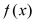objective function, which can be linear or non-linear,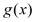linear or non-linear inequalities independent of the discrete choices,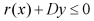mixed-integer inequalities that can contain linear or non-linear continuous terms,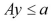Integer inequalities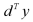fixed cost terms.